Exponents

Chapter 11 Class 7 Exponents and Powers
Concept wise

Suppose we have a number

128

To write it as exponent,

we do it’s prime factorization

128 = 2 × 2 × 2 × 2 × 2 × 2 × 2

128 = 2 7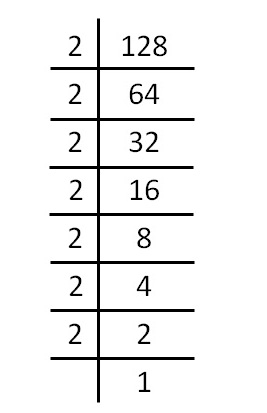Similarly,

For 243

243 = 3 × 3 × 3 × 3 × 3

243 = 3 5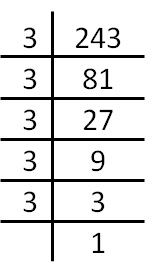For 625

625 = 5 × 5 × 5 × 5

625 = 5 4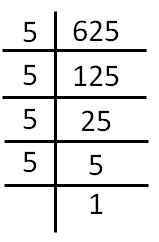For 18

18 = 2 × 3 × 3

18 = 2 × 3 2

For 72

72 = 2 × 2 × 2 × 3 × 3

72 = 2 3 × 3 2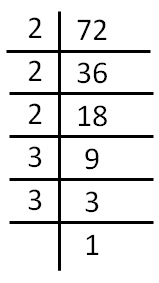For 100

100 = 2 × 2 × 5 × 5

100 = 2 2 × 5 2

We can also write 100 as

100 = 10 × 10 = 10 2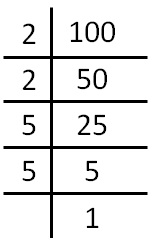Learn in your speed, with individual attention - Teachoo Maths 1-on-1 Class